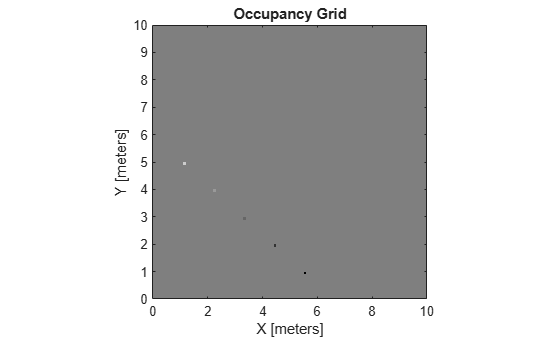# inflate

Inflate each occupied grid location

## Syntax

``inflate(map,radius)``
``inflate(map,gridradius,'grid')``

## Description

example

````inflate(map,radius)` inflates each occupied position of the specified `map` by the `radius`, specified in meters. Occupied location values are based on the `map.OccupiedThreshold` property. `radius` is rounded up to the nearest equivalent cell based on the resolution of the map. Values are modified using grayscale inflation to inflate higher probability values across the grid. This inflation increases the size of the occupied locations in the map.```
````inflate(map,gridradius,'grid')` inflates each occupied position by the `gridradius`, specified in number of cells.```

## Examples

collapse all

Create a 10 m-by-10 m empty map.

`map = occupancyMap(10,10,10);`

Update the occupancy of world locations with specific probability values and display the map.

```x = [1.2; 2.3; 3.4; 4.5; 5.6]; y = [5.0; 4.0; 3.0; 2.0; 1.0]; pvalues = [0.2 0.4 0.6 0.8 1]; updateOccupancy(map,[x y],pvalues) figure show(map)```Inflate occupied areas by a radius of 0.5 m. Larger occupancy values overwrite the smaller values.

```inflate(map,0.5) figure show(map)```Get grid locations from world locations.

`ij = world2grid(map,[x y]);`

Set grid locations to occupied locations.

```setOccupancy(map,ij,ones(5,1),'grid') figure show(map)```## Input Arguments

collapse all

Map representation, specified as a `occupancyMap` object. This object represents the environment of the vehicle. The object contains a matrix grid with values representing the probability of the occupancy of that cell. Values close to 1 represent a high probability that the cell contains an obstacle. Values close to 0 represent a high probability that the cell is not occupied and obstacle free.

Dimension that defines by how much to inflate occupied locations, specified as a scalar in meters. `radius` is rounded up to the nearest equivalent cell value.

Data Types: `double`

Number of cells by which to inflate the occupied locations, specified as a positive integer scalar.

Data Types: `double`

collapse all

### Grayscale Inflation

In grayscale inflation, the `strel` function creates a circular structuring element using the inflation radius. The grayscale inflation of A(x, y) by B(x, y) is defined as:

(AB)(x, y) = max {A(xx′, y’y′) +B(x', y') | (x′, y′) ∊ DB}.

DB is the domain of the probability values in the structuring element B. A(x,y) is assumed to be +∞ outside the domain of the grid.

Grayscale inflation acts as a local maximum operator and finds the highest probability values for nearby cells. The `inflate` method uses this definition to inflate the higher probability values throughout the grid. This inflation increases the size of any occupied locations and creates a buffer zone for vehicles to use as they navigate.